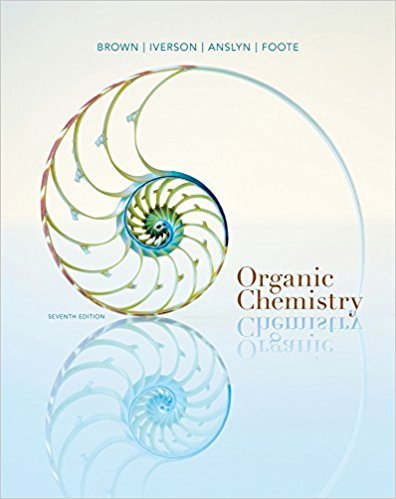×
Get Full Access to Organic Chemistry - 7 Edition - Chapter 2 - Problem 2.4
Get Full Access to Organic Chemistry - 7 Edition - Chapter 2 - Problem 2.4

×

# Combine the proper prefix, infix, and suffix and write the IUPAC name for eachcompoundISBN: 9781133952848 483

## Solution for problem 2.4 Chapter 2

Organic Chemistry | 7th Edition

• Textbook Solutions
• 2901 Step-by-step solutions solved by professors and subject experts
• Get 24/7 help from StudySoup virtual teaching assistantsOrganic Chemistry | 7th Edition

4 5 1 289 Reviews
21
0
Problem 2.4

Combine the proper prefix, infix, and suffix and write the IUPAC name for eachcompound.

Step-by-Step Solution:
Step 1 of 3

Review Test Submission: Practice Exam for Exam 1 – ... 2/14/17, 4:36 AM Three length scales ordered from smallest to largest are: Selected Answer: e.none of these. Answers: a. microscale, nanoscale, macroscale. b. microscale, macroscale, nanoscale. c. macroscale, nanoscale, microscale. d.nanoscale, microscale, macroscale. e. none of these. Question 3 1 out of 1 points 5.21 cm is the same distance as ______. Selected Answer: c.0.0521 m Answers: a. 5.21 mm b. 0.00521 km c.0.0521 m d. 5210 m e. 52.1 dm Question 4 0 out of 1 points Signiﬁcant ﬁgures are important because they indicate _______. Selected Answer: b.the accuracy of the conversion factor Answers: a.the number of digits in a measurement b. the accuracy of the conversion factor

Step 2 of 3

Step 3 of 3

## Discover and learn what students are asking

Calculus: Early Transcendental Functions : Inverse Trigonometric Functions: Integration
?In Exercises 1-20, find the indefinite integral. $$\int \frac{1}{4+(x-3)^{2}} d x$$

Calculus: Early Transcendental Functions : Second-Order Nonhomogeneous Linear Equations
?Verifying a Solution In Exercises 1-4,verify the solution of the differential equation. Solution

Chemistry: The Central Science : Liquids and Intermolecular Forces
?The phase diagram for neon is Use the phase diagram to answer the following

Statistics: Informed Decisions Using Data : The Binomial Probability Distribution
?State the criteria for a binomial probability experiment.

Unlock Textbook Solution